Theory and Modern Applications

# Approximate ∗-derivations on fuzzy Banach ∗-algebras

## Abstract

In this paper, we establish functional equations of -derivations and prove the stability of -derivations on fuzzy Banach -algebras. We also prove the superstability of -derivations on fuzzy Banach -algebras.

MSC:39B52, 47B47, 46L05, 39B72.

## 1 Introduction

Let $\mathcal{A}$ be a Banach -algebra. A linear mapping $\delta :D\left(\delta \right)\to \mathcal{A}$ is said to be a derivation on $\mathcal{A}$ if $\delta \left(ab\right)=\delta \left(a\right)b+a\delta \left(b\right)$ for all $a,b\in \mathcal{A}$, where $D\left(\delta \right)$ is a domain of δ and $D\left(\delta \right)$ is dense in $\mathcal{A}$. If δ satisfies the additional condition $\delta \left({a}^{\ast }\right)=\delta {\left(a\right)}^{\ast }$ for all $a\in \mathcal{A}$, then δ is called a -derivation on $\mathcal{A}$. It is well known that if $\mathcal{A}$ is a ${C}^{\ast }$-algebra and $D\left(\delta \right)$ is A, then the -derivation δ is bounded. For several reasons, the theory of bounded derivations of ${C}^{\ast }$-algebras is very important in the theory of quantum mechanics and operator algebras [3, 4].

A functional equation is called stable if any function satisfying a functional equation “approximately” is near to a true solution of the functional equation. We say that a functional equation is superstable if every approximate solution is an exact solution of it.

In 1940, Ulam  proposed the following question concerning stability of group homomorphisms: Under what condition is there an additive mapping near an approximately additive mapping? Hyers  answered positively the problem of Ulam for the case where ${G}_{1}$ and ${G}_{2}$ are Banach spaces. A generalized version of the theorem of Hyers for an approximately linear mapping was given by ThM Rassias . Since then, the stability problems of various functional equations have been extensively investigated by a number of authors (for instances, [1, 2, 9, 10, 19, 20]). In particular, those of the important functional equations are the following functional equations: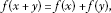(1.1)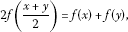(1.2)

which are called the Cauchy equation and the Jensen equation, respectively. Every solution of the functional equations (1.1) and (1.2) is said to be an additive mapping.

Since Katsaras  introduced the idea of fuzzy norm on a linear space, several definitions for a fuzzy norm on a linear space have been introduced and discussed from different points of view . We use the definition of fuzzy normed spaces given in [5, 17] to investigate the stability of derivation in the fuzzy Banach -algebra setting. The stability of functional equations in fuzzy normed spaces was begun by , after then lots of results of fuzzy stability were investigated [11, 13, 16, 18].

Definition 1.1 [5, 17, 21]

Let X be a real vector space. A function $N:X×\mathbb{R}\to \left[0,1\right]$ is called a fuzzy norm on X if for all $x,y\in X$ and all $s,t\in \mathbb{R}$,

(${N}_{1}$) $N\left(x,t\right)=0$ for $t\le 0$;

(${N}_{2}$) $x=0$ if and only if $N\left(x,t\right)=1$ for all $t>0$;

(${N}_{3}$) $N\left(cx,t\right)=N\left(x,\frac{t}{|c|}\right)$ if $c\ne 0$;

(${N}_{4}$) $N\left(x+y,s+t\right)\ge min\left\{N\left(x,s\right),N\left(y,t\right)\right\}$;

(${N}_{5}$) $N\left(x,\cdot \right)$ is a non-decreasing function of $\mathbb{R}$ and ${lim}_{t\to \mathrm{\infty }}N\left(x,t\right)=1$;

(${N}_{6}$) for $x\ne 0$, $N\left(x,\cdot \right)$ is continuous on $\mathbb{R}$.

The pair $\left(X,N\right)$ is called a fuzzy normed vector space.

Furthermore, we can make $\left(X,N\right)$ a fuzzy normed -algebra if we add (${N}_{7}$) and (${N}_{8}$) as follows:

(${N}_{7}$) $N\left(xy,st\right)\ge min\left\{N\left(x,s\right),N\left(y,t\right)\right\}$;

(${N}_{8}$) $N\left(x,t\right)=N\left({x}^{\ast },t\right)$.

The properties and examples of fuzzy normed vector spaces, fuzzy algebras, and fuzzy norms are given in [17, 18, 22, 23].

Definition 1.2 [5, 17, 21]

Let $\left(X,N\right)$ be a fuzzy normed vector space. A sequence $\left\{{x}_{n}\right\}$ in X is said to be convergent or converge if there exists an $x\in X$ such that ${lim}_{n\to \mathrm{\infty }}N\left({x}_{n}-x,t\right)=1$ for all $t>0$. In this case, x is called the limit of the sequence $\left\{{x}_{n}\right\}$ and we denote it by N-${lim}_{n\to \mathrm{\infty }}{x}_{n}=x$.

Definition 1.3 [5, 17, 21]

Let $\left(X,N\right)$ be a fuzzy normed vector space. A sequence $\left\{{x}_{n}\right\}$ in X is called Cauchy if for each $\epsilon >0$ and each $t>0$ there exists an ${n}_{0}\in \mathbb{N}$ such that for all $n\ge {n}_{0}$ and all $p>0$, we have $N\left({x}_{n+p}-{x}_{n},t\right)>1-\epsilon$.

It is well known that every convergent sequence in a fuzzy normed vector space is Cauchy. If each Cauchy sequence is convergent, then the fuzzy norm is said to be complete and the fuzzy normed vector space is called a fuzzy Banach space.

We say that a mapping $f:X\to Y$ between fuzzy normed vector spaces X and Y is continuous at a point ${x}_{0}\in X$ if for each sequence $\left\{{x}_{n}\right\}$ converging to ${x}_{0}$ in X, then the sequence $\left\{f\left({x}_{n}\right)\right\}$ converges to $f\left({x}_{0}\right)$. If $f:X\to Y$ is continuous at each $x\in X$, then $f:X\to Y$ is said to be continuous on X.

In this paper, using the functional equation of -derivations

$f\left(\lambda a+b+cd\right)=\lambda f\left(a\right)+f\left(b\right)+f\left(c\right)d+cf\left(d\right)$

introduced in  we prove fuzzy version of the stability of -derivations associated to the Cauchy functional equation and the Jensen functional equation. We also prove the superstability of -derivations on fuzzy Banach -algebras.

## 2 Stability of ∗-derivations on fuzzy Banach ∗-algebras

In this section, let $\mathcal{A}$ be a fuzzy Banach -algebra.

Theorem 2.1 Let $\phi :{\mathcal{A}}^{4}\to \left[0,\mathrm{\infty }\right)$ and $\psi :{\mathcal{A}}^{2}\to \left[0,\mathrm{\infty }\right)$ be control functions such that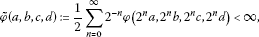(2.1)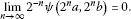(2.2)

Suppose that $f:\mathcal{A}\to \mathcal{A}$ is a mapping with $f\left(0\right)=0$ satisfying the followings:

$\underset{t\to \mathrm{\infty }}{lim}N\left(f\left(\lambda a+b+cd\right)-\lambda f\left(a\right)-f\left(b\right)-f\left(c\right)d-cf\left(d\right),t\phi \left(a,b,c,d\right)\right)=1$
(2.3)

uniformly on ${\mathcal{A}}^{4}$ and for all $\lambda \in \mathbb{T}:=\left\{\lambda \in \mathbb{C}:|\lambda |=1\right\}$

$\underset{t\to \mathrm{\infty }}{lim}N\left(f{\left(a\right)}^{\ast }-f\left({a}^{\ast }\right),t\psi \left(a,{a}^{\ast }\right)\right)=1$
(2.4)

uniformly on ${\mathcal{A}}^{2}$. Then there exists a unique -derivation δ on $\mathcal{A}$ satisfying

$\underset{t\to \mathrm{\infty }}{lim}N\left(f\left(a\right)-\delta \left(a\right),t\stackrel{˜}{\phi }\left(a,a,0,0\right)\right)=1$
(2.5)

for all $a\in \mathcal{A}$.

Proof Let $0<ϵ<1$ be given. Setting $a=b$, $c=d=0$ and $\lambda =1$ in (2.3), we can find some ${t}_{0}>0$ such that

$N\left(f\left(2a\right)-2f\left(a\right),t\phi \left(a,a,0,0\right)\right)\ge 1-ϵ$

for all $a\in \mathcal{A}$ and $t\ge {t}_{0}$. One can use induction to show that

$N\left(f\left({2}^{n}a\right)-{2}^{n}f\left(a\right),t\sum _{k=0}^{n-1}{2}^{n-k-1}\phi \left({2}^{k}a,{2}^{k}a,0,0\right)\right)\ge 1-ϵ.$
(2.6)

Let $t={t}_{0}$ and put $n=p$ then by replacing a with ${2}^{n}a$ in (2.6), we obtain

$N\left(\frac{f\left({2}^{n+p}a\right)}{{2}^{n+p}}-\frac{f\left({2}^{n}a\right)}{{2}^{n}},\frac{{t}_{0}}{{2}^{n+p}}\sum _{k=0}^{p-1}{2}^{p-k-1}\phi \left({2}^{n+k}a,{2}^{n+k}a,0,0\right)\right)\ge 1-ϵ$
(2.7)

for all integers $n\ge 0$, $p\ge 0$. By the convergence of (2.1) there is ${n}_{0}\in \mathbb{N}$ such that

$\frac{{t}_{0}}{2}\sum _{k=n}^{n+p-1}{2}^{-k}\phi \left({2}^{k}a,{2}^{k}a,0,0\right)\le \delta$

for all $n\ge {n}_{0}$ and $p>0$. Since the fuzzy norm $N\left(x,\cdot \right)$ is nondecreasing, we can have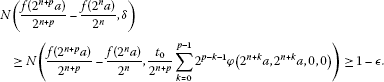(2.8)

It follows from (2.8) and Definition 1.3 that the sequence $\left\{\frac{f\left({2}^{n}a\right)}{{2}^{n}}\right\}$ is Cauchy. Due to the completeness of $\mathcal{A}$, this sequence is convergent. Define

$\delta \left(a\right):=N-\underset{n\to \mathrm{\infty }}{lim}\frac{f\left({2}^{n}a\right)}{{2}^{n}}$
(2.9)

for all $a\in \mathcal{A}$. From the above equation, we have

$\delta \left(\frac{1}{{2}^{k}}a\right)=N-\underset{n\to \mathrm{\infty }}{lim}\frac{1}{{2}^{k}}\frac{f\left({2}^{n-k}a\right)}{{2}^{n-k}}=\frac{1}{{2}^{k}}\delta \left(a\right)$
(2.10)

for each $k\in \mathbb{N}$. Moreover, letting $n=0$ and passing the limit $p\to \mathrm{\infty }$ in (2.8), we get

$\underset{t\to \mathrm{\infty }}{lim}N\left(f\left(a\right)-\delta \left(a\right),t\stackrel{˜}{\phi }\left(a,a,0,0\right)\right)=1$
(2.11)

for all $a\in \mathcal{A}$. Putting $c=d=0$ and replacing a and b by ${2}^{n}a$ and ${2}^{n}b$, respectively, in (2.3), there exists ${t}_{0}>0$ such that

$N\left({2}^{-n}f\left({2}^{n}\left(\lambda a+b\right)\right)-\lambda {2}^{-n}f\left({2}^{n}a\right)-{2}^{-n}f\left({2}^{n}b\right),t{2}^{-n}\phi \left({2}^{n}a,{2}^{n}b,0,0\right)\right)\ge 1-ϵ$

for all $t\ge {t}_{0}$. Let $a,b\in \mathcal{A}$. Temporarily fix $t>0$. Since ${lim}_{n\to \mathrm{\infty }}\frac{1}{{2}^{n}}t\phi \left({2}^{n}a,{2}^{n}b,0,0\right)=0$, there exists ${n}_{0}>0$ such that

$t\phi \left({2}^{n}a,{2}^{n}a,0,0\right)\le \frac{{2}^{n}t}{4},$

for all $n\ge {n}_{0}$. Hence, we have

$\begin{array}{c}N\left(\delta \left(\lambda a+b\right)-\lambda \delta \left(a\right)-\delta \left(b\right),t\right)\hfill \\ \phantom{\rule{1em}{0ex}}\ge min\left\{N\left(\delta \left(\lambda a+b\right)-{2}^{-n}f\left({2}^{n}\left(\lambda a+b\right)\right),\frac{t}{4}\right),N\left(\lambda \delta \left(a\right)-\lambda {2}^{-n}f\left({2}^{n}a\right),\frac{t}{4}\right),\hfill \\ \phantom{\rule{2em}{0ex}}N\left(\delta \left(b\right)-{2}^{-n}f\left({2}^{n}b\right),\frac{4}{t}\right),N\left(f\left({2}^{n}\left(\lambda a+b\right)\right)-\lambda f\left({2}^{n}a\right)-f\left({2}^{n}b\right),\frac{{2}^{n}4}{t}\right)\right\}\hfill \end{array}$

for all $n\ge {n}_{0}$ and $t>0$. The first three terms on the second and third lines of the above inequality tend to 1 as $n\to \mathrm{\infty }$. Furthermore, the last term is greater than

$N\left(f\left({2}^{n}\left(\lambda a+b\right)\right)-\lambda f\left({2}^{n}a\right)-f\left({2}^{n}b\right),{t}_{0}\phi \left({2}^{n}a,{2}^{n}b,0,0\right)\right),$

which is greater than or equal to $1-ϵ$. Therefore,

$N\left(\delta \left(\lambda a+b\right)-\lambda \delta \left(a\right)-\delta \left(b\right),t\right)\ge 1-ϵ$

for all $t>0$. It follows that $\delta \left(\lambda a+b\right)=\lambda \delta \left(a\right)+\delta \left(b\right)$ by (${N}_{2}$) for all $a,b\in \mathcal{A}$ and all $\lambda \in \mathbb{T}$. Next, let $\lambda ={\lambda }_{1}+\text{i}{\lambda }_{2}\in \mathbb{C}$ where ${\lambda }_{1},{\lambda }_{2}\in \mathbb{R}$. Let ${\gamma }_{1}={\lambda }_{1}-\left[{\lambda }_{1}\right]$ and ${\gamma }_{2}={\lambda }_{2}-\left[{\lambda }_{2}\right]$, where $\left[\lambda \right]$ denotes the integer part of λ. Then $0\le {\gamma }_{i}<1$ ($1\le i\le 2$). One can represent ${\gamma }_{i}$ as ${\gamma }_{i}=\frac{{\lambda }_{i,1}+{\lambda }_{i,2}}{2}$ such that ${\lambda }_{i,j}\in \mathbb{T}$ ($1\le i$, $j\le 2$). From (2.10), we infer that

for all $x\in \mathcal{A}$. Hence, δ is $\mathbb{C}$-linear. Putting $a=b=0$ and replacing c and d by ${2}^{n}c$ and ${2}^{n}d$, respectively, in (2.3), there exists ${t}_{0}>0$ such that

$N\left({2}^{-2n}f\left({2}^{2n}cd\right)-{2}^{-2n}f\left({2}^{n}c\right)\left({2}^{n}d\right)-{2}^{-2n}\left({2}^{n}c\right)f\left({2}^{n}d\right),t{2}^{-2n}\phi \left(0,0,{2}^{n}c,{2}^{n}d\right)\right)\ge 1-ϵ$

for all $t\ge {t}_{0}$. Fix $t\left(>0\right)$ temporarily. By (2.1) there exists ${n}_{0}>0$ such that

$t\phi \left(0,0,{2}^{n}c,{2}^{n}d\right)\le \frac{{2}^{2n}t}{4}$

for all $n\ge {n}_{0}$ and $t>0$. We have

$\begin{array}{c}N\left(\delta \left(cd\right)-\delta \left(c\right)d-c\delta \left(d\right),t\right)\hfill \\ \phantom{\rule{1em}{0ex}}\ge min\left\{N\left(\delta \left(cd\right)-{2}^{-2n}f\left({2}^{2n}cd\right),\frac{t}{4}\right),N\left(\delta \left(c\right)d-{2}^{-2n}f\left({2}^{n}c\right)\left({2}^{n}d\right),\frac{t}{4}\right),\hfill \\ \phantom{\rule{2em}{0ex}}N\left(c\delta \left(d\right)-{2}^{-2n}\left({2}^{n}c\right)f\left({2}^{n}d\right),\frac{t}{4}\right),\hfill \\ \phantom{\rule{2em}{0ex}}N\left(f\left({2}^{2n}cd\right)-f\left({2}^{n}c\right)\left({2}^{n}d\right)-\left({2}^{n}c\right)f\left({2}^{n}d\right),\frac{{2}^{2n}4}{t}\right)\right\}\hfill \\ \phantom{\rule{1em}{0ex}}\ge min\left\{N\left(\delta \left(cd\right)-{2}^{-2n}f\left({2}^{2n}cd\right),\frac{t}{4}\right),N\left(\delta \left(c\right)d-{2}^{-2n}f\left({2}^{n}c\right)\left({2}^{n}d\right),\frac{t}{4}\right),\hfill \\ \phantom{\rule{2em}{0ex}}N\left(c\delta \left(d\right)-{2}^{-2n}\left({2}^{n}c\right)f\left({2}^{n}d\right),\frac{t}{4}\right),\hfill \\ \phantom{\rule{2em}{0ex}}N\left(f\left({2}^{2n}cd\right)-f\left({2}^{n}c\right)\left({2}^{n}d\right)-\left({2}^{n}c\right)f\left({2}^{n}d\right),t\phi \left(0,0,{2}^{n}c,{2}^{n}d\right)\right)\right\}\hfill \end{array}$

for all $n\ge {n}_{0}$ and $t>0$. From the above computation

$\delta \left(cd\right)=\delta \left(c\right)d+c\delta \left(d\right)$
(2.12)

for all $c,d\in \mathcal{A}$. So it is a derivation on $\mathcal{A}$. Moreover, it follows from (2.7) with $n=0$ and (2.9) that ${lim}_{t\to \mathrm{\infty }}N\left(\delta \left(a\right)-f\left(a\right),t\stackrel{˜}{\phi }\left(a,a,0,0\right)\right)=1$ for all $a\in \mathcal{A}$. It is well known that the additive mapping δ satisfying (2.5) is unique (see  or ). Replacing a and ${a}^{\ast }$ by ${2}^{n}a$ and ${2}^{n}{a}^{\ast }$, respectively, in (2.4) we can find ${t}_{0}>0$ such that

$N\left({2}^{-n}f{\left({2}^{n}a\right)}^{\ast }-{2}^{-n}f\left({2}^{n}{a}^{\ast }\right),t{2}^{-n}\psi \left({2}^{n}a,{2}^{n}{a}^{\ast }\right)\right)\ge 1-ϵ$

for all $a\in \mathcal{A}$ and all $t>{t}_{0}$. Since ${lim}_{n\to \mathrm{\infty }}{2}^{-n}\psi \left({2}^{n}a,{2}^{n}{a}^{\ast }\right)=0$, there exists some ${n}_{0}>0$ such that $t\psi \left({2}^{n}a,{2}^{n}{a}^{\ast }\right)<\frac{t{2}^{n}}{2}$ for all $n\ge {n}_{0}$. Hence,

$\begin{array}{c}N\left(\delta {\left(a\right)}^{\ast }-\delta \left({a}^{\ast }\right),t\right)\hfill \\ \phantom{\rule{1em}{0ex}}\ge min\left\{N\left(\delta {\left(a\right)}^{\ast }-{2}^{-n}f{\left({2}^{n}a\right)}^{\ast },\frac{t}{4}\right),N\left(\delta \left({a}^{\ast }\right)-{2}^{-n}f\left({2}^{n}{a}^{\ast }\right),\frac{t}{4}\right),\hfill \\ \phantom{\rule{2em}{0ex}}N\left(f{\left({2}^{n}a\right)}^{\ast }-f\left({2}^{n}{a}^{\ast }\right),\frac{{2}^{n}t}{2}\right)\right\}.\hfill \end{array}$

The first two terms on the right-hand side of the above inequality tend to 1 as $n\to \mathrm{\infty }$. Furthermore, the last term is greater than

$N\left(f{\left({2}^{n}a\right)}^{\ast }-f\left({2}^{n}{a}^{\ast }\right),t\psi \left({2}^{n}a,{2}^{n}{a}^{\ast }\right)\right),$

which is greater than or equal to $1-ϵ$. So, we have that $N\left(\delta {\left(a\right)}^{\ast }-\delta \left({a}^{\ast }\right),t\right)>1-ϵ$ for all $t>0$. It follows from that $\delta \left({a}^{\ast }\right)=\delta {\left(a\right)}^{\ast }$ for all $a\in \mathcal{A}$. So, δ is a *-derivation on $\mathcal{A}$. □

Theorem 2.2 Suppose that $f:\mathcal{A}\to \mathcal{A}$ is a mapping with $f\left(0\right)=0$ for which there exist functions $\phi :{\mathcal{A}}^{4}\to \left[0,\mathrm{\infty }\right)$ and $\psi :{\mathcal{A}}^{2}\to \left[0,\mathrm{\infty }\right)$ such that

$\begin{array}{c}\stackrel{˜}{\phi }\left(a,b,c,d\right):=\frac{1}{2}\sum _{n=0}^{\mathrm{\infty }}{2}^{n}\phi \left({2}^{-n}a,{2}^{-n}b,{2}^{-n}c,{2}^{-n}d\right)<\mathrm{\infty },\hfill \\ \underset{n\to \mathrm{\infty }}{lim}{2}^{n}\psi \left({2}^{-n}a,{2}^{-n}b\right)=0,\hfill \\ \underset{t\to \mathrm{\infty }}{lim}N\left(f\left(\lambda a+b+cd\right)-\lambda f\left(a\right)-f\left(b\right)-f\left(c\right)d-cf\left(d\right),t\phi \left(a,b,c,d\right)\right)=1,\hfill \\ \underset{t\to \mathrm{\infty }}{lim}N\left(f{\left(a\right)}^{\ast }-f{\left(a\right)}^{\ast },t\psi \left(a,{a}^{\ast }\right)\right)=1\hfill \end{array}$

for all $\lambda \in \mathbb{T}$ and all $a,b,c,d\in \mathcal{A}$. Then there exists a unique -derivation δ on $\mathcal{A}$ satisfying

$\underset{t\to \mathrm{\infty }}{lim}N\left(f\left(a\right)-\delta \left(a\right),t\stackrel{˜}{\phi }\left(a,a,0,0\right)\right)=1$

for all $a\in \mathcal{A}$.

## 3 Stability of ∗-derivations associated to the Jensen equation

The stability of the Jensen equation has been studied first by Kominek and then by several other mathematicians: (). In this section, we study the stability of -derivation associated to the Jensen equation in a fuzzy Banach -algebra $\mathcal{A}$.

Theorem 3.1 Let $\mathcal{A}$ be a fuzzy Banach -algebra. Suppose that $f:\mathcal{A}\to \mathcal{A}$ is a mapping with $f\left(0\right)=0$ for which there exist functions $\phi :{\mathcal{A}}^{2}\to \left[0,\mathrm{\infty }\right)$ and ${\psi }_{i}:{\mathcal{A}}^{2}\to \left[0,\mathrm{\infty }\right)$ ($1\le i\le 2$) such that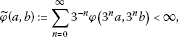(3.1)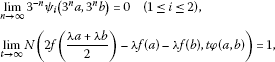(3.2)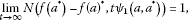(3.3)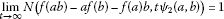(3.4)

for all $a,b\in \mathcal{A}$ and all $\lambda \in \mathbb{T}$. Then there exists a unique -derivation δ on $\mathcal{A}$ satisfying

$\underset{t\to \mathrm{\infty }}{lim}N\left(f\left(a\right)-\delta \left(a\right),\frac{t}{3}\left(\stackrel{˜}{\phi }\left(a,-a\right)+\stackrel{˜}{\phi }\left(-a,3a\right)\right)\right)=1$
(3.5)

for all $a\in \mathcal{A}$.

Proof Let $0<ϵ<1$ be given. Letting $\lambda =-1$ and $b=-a$ in (3.2), we can find some ${t}_{0}>0$ such that

$N\left(f\left(a\right)+f\left(-a\right),t\phi \left(a,-a\right)\right)\ge 1-ϵ$

for all $a\in \mathcal{A}$ and $t\ge {t}_{0}$. Letting $\lambda =1$ and replacing a and b by −a and 3a, respectively, in (3.2), we get also ${t}_{1}\ge {t}_{0}$ such that

$N\left(2f\left(a\right)-f\left(-a\right)-f\left(3a\right),t\phi \left(-a,3a\right)\right)\ge 1-ϵ$

for all $a\in \mathcal{A}$ and $t\ge {t}_{1}$. Thus,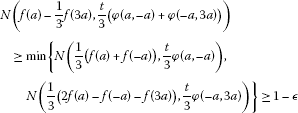(3.6)

for all $a\in \mathcal{A}$. Replace a by ${3}^{n}a$ in (3.6)

$N\left(\frac{f\left({3}^{n}a\right)}{{3}^{n}}-\frac{f\left({3}^{n+1}a\right)}{{3}^{n+1}},\frac{t}{{3}^{n+1}}\left(\phi \left({3}^{n}a,-{3}^{n}a\right)+\phi \left(-{3}^{n}a,{3}^{n+1}a\right)\right)\right)\ge 1-ϵ.$

Given $\delta >0$, there exists an integer ${n}_{0}>0$ such that

$\frac{t}{3}\sum _{j=m}^{n-1}{3}^{-j}\left(\phi \left({3}^{j}a,-{3}^{j}a\right)+\phi \left(-{3}^{j}a,{3}^{j+1}a\right)\right)\le \delta$

for all $n\ge m\ge {n}_{0}$.

So, we have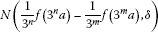(3.7)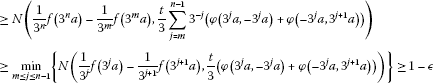(3.8)

for all nonnegative integers n, m with $n\ge m\ge {n}_{0}$ and all $a\in \mathcal{A}$. It follows from Definition 1.3 that the sequence $\left\{\frac{1}{{3}^{n}}f\left({3}^{n}a\right)\right\}$ is a Cauchy sequence for all $a\in \mathcal{A}$. Since $\mathcal{A}$ is complete, the sequence $\left\{\frac{1}{{3}^{n}}f\left({3}^{n}a\right)\right\}$ is convergent. So, one can define the mapping $\delta :\mathcal{A}\to \mathcal{A}$ by

$\delta \left(a\right)=N-\underset{n\to \mathrm{\infty }}{lim}\frac{1}{{3}^{n}}f\left({3}^{n}a\right)$
(3.9)

for all $a\in \mathcal{A}$. If we put $\lambda =1$ and replace a, b with ${3}^{n}a$, ${3}^{n}b$, respectively, in (3.2), we can find some ${t}_{0}>0$ such that

$N\left(2f\left({3}^{n}\frac{a+b}{2}\right)-f\left({3}^{n}a\right)-f\left({3}^{n}b\right),{3}^{-n}t\phi \left({3}^{n}a,{3}^{n}b\right)\right)\ge 1-ϵ$

for all $t\ge {t}_{0}$. Fix $t>0$ temporarily. Since ${lim}_{n\to \mathrm{\infty }}{3}^{-n}\phi \left({3}^{n}a,{3}^{n}b\right)=0$, there is some ${n}_{0}>0$ such that $t\phi \left({3}^{n}a,{3}^{n}b\right)<\frac{{3}^{n}t}{4}$ for all $n\ge {n}_{0}$. Then we have

$\begin{array}{c}N\left(2\delta \left(\frac{a+b}{2}\right)-\delta \left(a\right)-\delta \left(b\right),t\right)\hfill \\ \phantom{\rule{1em}{0ex}}\ge min\left\{N\left(2\delta \left(\frac{a+b}{2}\right)-\frac{1}{{3}^{n}}2f\left({3}^{n}\frac{a+b}{2}\right),\frac{t}{4}\right),N\left(\delta \left(a\right)-\frac{f\left({3}^{n}a\right)}{{3}^{n}},\frac{t}{4}\right),\hfill \\ \phantom{\rule{2em}{0ex}}N\left(\delta \left(b\right)-\frac{f\left({3}^{n}b\right)}{{3}^{n}},\frac{t}{4}\right),N\left(2f\left({3}^{n}\frac{a+b}{2}\right)-f\left({3}^{n}a\right)-f\left({3}^{n}b\right),\frac{{3}^{n}t}{4}\right)\right\}\hfill \end{array}$

for all $a,b\in \mathcal{A}$ and $t>0$. The first three terms on the second and third lines of the above inequality tend to 1 as $n\to \mathrm{\infty }$. Furthermore, the last term is greater than

$N\left(2f\left({3}^{n}\frac{a+b}{2}\right)-f\left({3}^{n}a\right)-f\left({3}^{n}b\right),t\phi \left({3}^{n}a,{3}^{n}b\right)\right),$

which is greater than or equal to $1-ϵ$.

So, we have

$N\left(2\delta \left(\frac{a+b}{2}\right)-\delta \left(a\right)-\delta \left(b\right),t\right)\ge 1-ϵ$

for all $t>0$. By the definition of fuzzy norm, we have

$2\delta \left(\frac{a+b}{2}\right)=\delta \left(a\right)+\delta \left(b\right)$
(3.10)

for all $a,b\in \mathcal{A}$. Since $f\left(0\right)=0$, we have $\delta \left(0\right)=0$. Putting $b=0$ in (3.10), we get $2\delta \left(\frac{a}{2}\right)=\delta \left(a\right)$ for each $a\in \mathcal{A}$ and, therefore, $\delta \left(a\right)+\delta \left(b\right)=2\delta \left(\frac{a+b}{2}\right)=\delta \left(a+b\right)$ for all $a,b\in \mathcal{A}$. Moreover, letting $m=0$ and passing the limit $n\to \mathrm{\infty }$ in (3.8), we get

$N\left(f\left(a\right)-\delta \left(a\right),\frac{t}{3}\left(\stackrel{˜}{\phi }\left(a,-a\right)+\stackrel{˜}{\phi }\left(-a,3a\right)\right)\right)\ge 1-ϵ$

for all $a\in \mathcal{A}$. So, we have Eq. (3.5). It is known that such an additive mapping δ is unique. Let $\lambda \in \mathbb{T}$. Replacing both a and b in (3.2) by ${3}^{n}a$ and dividing the both sides of the obtained inequality by ${3}^{n}$, there exists some ${t}_{0}>0$ such that

$N\left({3}^{-n}f\left(\lambda {3}^{n}a\right)-\lambda {3}^{-n}f\left({3}^{n}a\right),{3}^{-n}t\phi \left({3}^{n}a,{3}^{n}a\right)\right)\ge 1-ϵ$

for all $a\in \mathcal{A}$ and all $t\ge {t}_{0}$. Fix $t>0$ temporarily. Since ${lim}_{n\to \mathrm{\infty }}{3}^{-n}\varphi \left({3}^{n}a,{3}^{n}b\right)=0$, there exists ${n}_{0}>0$ such that ${3}^{-n}\varphi \left({3}^{n}a,{3}^{n}b\right)\le \frac{t}{2}$ for all $n\ge {n}_{0}$.

If we consider the following inequality

$\begin{array}{c}N\left(\delta \left(\lambda a\right)-\lambda \delta \left(a\right),t\right)\hfill \\ \phantom{\rule{1em}{0ex}}\ge min\left\{N\left(\delta \left(\lambda a\right)-{3}^{-n}f\left(\lambda {3}^{n}a\right),\frac{t}{4}\right),N\left(\lambda \delta \left(a\right)-{3}^{-n}f\left(\lambda {3}^{n}a\right),\frac{t}{4}\right),\hfill \\ \phantom{\rule{2em}{0ex}}N\left({3}^{-n}f\left(\lambda {3}^{n}a\right)-{3}^{-n}f\left(\lambda {3}^{n}a\right),\frac{t}{2}\right)\right\},\hfill \end{array}$

then the first two terms on the second line of the above inequality tend to 1 as $n\to \mathrm{\infty }$ and the last term is greater than

$N\left({3}^{-n}f\left(\lambda {3}^{n}a\right)-\lambda {3}^{-n}f\left({3}^{n}a\right),{3}^{-n}t\phi \left({3}^{n}a,{3}^{n}a\right)\right),$

which is greater than or equal to $1-ϵ$. So, we can get $\delta \left(\lambda a\right)=\lambda \delta \left(a\right)$ for all $\lambda \in \mathbb{C}$ by the similar discussion in the proof Theorem 2.1. Replacing both a and ${a}^{\ast }$ in (3.3) by ${3}^{n}a$ and ${3}^{n}{a}^{\ast }$, and then dividing the both sides of the obtained inequality by ${3}^{n}$, we find some ${t}_{0}>0$ such that

$N\left({3}^{-n}f{\left({3}^{n}a\right)}^{\ast }-{3}^{-n}f\left({3}^{n}{a}^{\ast }\right),t{3}^{-n}{\psi }_{1}\left({3}^{n}a,{3}^{n}{a}^{\ast }\right)\right)\ge 1-ϵ$

for all $t\ge {t}_{0}$. Fix $t>0$ temporarily. Since ${lim}_{n\to \mathrm{\infty }}{3}^{-n}{\psi }_{1}\left({3}^{n}a,{3}^{n}{a}^{\ast }\right)=0$, there exists ${n}_{0}>0$ such that ${3}^{-n}t{\psi }_{1}\left({3}^{n}a,{3}^{n}{a}^{\ast }\right)\le \frac{t}{2}$ for all $n\ge {n}_{0}$. We consider the following inequality:

$\begin{array}{c}N\left(\delta \left({a}^{\ast }\right)-\delta {\left(a\right)}^{\ast },t\right)\hfill \\ \phantom{\rule{1em}{0ex}}\ge min\left\{N\left(\delta \left({a}^{\ast }\right)-{3}^{-n}f\left({3}^{n}{a}^{\ast }\right),\frac{t}{4}\right),N\left(\delta {\left(a\right)}^{\ast }-{3}^{-n}f{\left({3}^{n}a\right)}^{\ast },\frac{t}{4}\right),\hfill \\ \phantom{\rule{2em}{0ex}}N\left({3}^{-n}f\left({3}^{n}{a}^{\ast }\right)-{3}^{-n}f{\left({3}^{n}a\right)}^{\ast },\frac{t}{2}\right)\right\}.\hfill \end{array}$

Then we get $\delta \left({a}^{\ast }\right)=\delta {\left(a\right)}^{\ast }$ for all $a\in \mathcal{A}$. For the derivation property, replacing both a and b in (3.4) by ${3}^{n}a$ and ${3}^{n}b$, we can find some ${t}_{0}>0$ such that

$N\left(\frac{f\left({3}^{2n}ab\right)}{{3}^{2n}}-\frac{{3}^{n}af\left({3}^{n}b\right)}{{3}^{2n}}-\frac{f\left({3}^{n}a\right)\left({3}^{n}b\right)}{{3}^{2n}},{3}^{-n}t{\psi }_{2}\left({3}^{n}a,{3}^{n}b\right)\right)\ge 1-ϵ$

for all $t\ge {t}_{0}$. By (3.4), there exists ${n}_{0}\in \mathbf{N}$ such that ${3}^{-n}t{\psi }_{2}\left({3}^{n}a,{3}^{n}b\right)\le \frac{t}{4}$ for all $n\ge {n}_{0}$ and $t>0$. We can get $\delta \left(ab\right)=\delta \left(a\right)b+a\delta \left(b\right)$ for all $a,b\in \mathcal{A}$ from the following computation:

$\begin{array}{c}N\left(\delta \left(ab\right)-a\delta \left(b\right)-\delta \left(a\right)b,t\right)\hfill \\ \phantom{\rule{1em}{0ex}}\ge min\left\{N\left(\delta \left(ab\right)-\frac{f\left({3}^{2n}ab\right)}{{3}^{2n}},\frac{t}{4}\right),N\left(a\delta \left(b\right)-\frac{{3}^{n}af\left({3}^{n}b\right)}{{3}^{2n}},\frac{t}{4}\right),\hfill \\ \phantom{\rule{2em}{0ex}}N\left(\delta \left(a\right)b-\frac{f\left({3}^{n}a\right)\left({3}^{n}b\right)}{{3}^{2n}},\frac{t}{4}\right),N\left(\frac{f\left({3}^{2n}ab\right)}{{3}^{2n}}-\frac{{3}^{n}af\left({3}^{n}b\right)}{{3}^{2n}}-\frac{f\left({3}^{n}a\right)\left({3}^{n}b\right)}{{3}^{2n}},\frac{t}{4}\right)\right\}.\hfill \end{array}$

Hence, δ is the -derivation on $\mathcal{A}$ that we want. □

## 4 Superstability of ∗-derivations

In this section, we prove the superstability of -derivations on a fuzzy Banach -algebras. More precisely, we introduce the concept of $\left(\psi ,\phi \right)$-approximate -derivation and show that any $\left(\psi ,\phi \right)$-approximate -derivation is just a -derivation.

Definition 4.1 Suppose that $\mathcal{A}$ is a -normed algebra and $s\in \left\{-1,1\right\}$. Let $\delta :\mathcal{A}\to \mathcal{A}$ be a mapping for which there exist a function $\phi :\mathcal{A}\to \mathcal{A}$, and functions ${\psi }_{i}:\mathcal{A}×\mathcal{A}\to \mathbb{R}$ ($1\le i\le 3$) satisfying

$\underset{n\to \mathrm{\infty }}{lim}{n}^{-s}{\psi }_{i}\left({n}^{s}a,b\right)=\underset{n\to \mathrm{\infty }}{lim}{n}^{-s}{\psi }_{i}\left(a,{n}^{s}b\right)=0\phantom{\rule{1em}{0ex}}\left(a,b\in \mathcal{A}\right)$
(4.1)

such that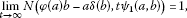(4.2)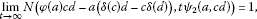(4.3)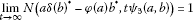(4.4)

for all $a,b,c,d\in \mathcal{A}$. Then δ is called a $\left(\psi ,\phi \right)$-approximate -derivation on $\mathcal{A}$.

Theorem 4.2 Let $\mathcal{A}$ be a fuzzy Banach -algebra with approximate unit. Then any $\left(\psi ,\phi \right)$-approximate -derivation δ on $\mathcal{A}$ is a -derivation.

Proof We assume that (4.1) holds. An arbitrary $ϵ>0$ is given. Let $a,b\in \mathcal{A}$ and $\lambda \in \mathbb{C}$. For $n\in \mathbb{N}$ there exists ${t}_{0}>0$ by (4.2) such that

$\begin{array}{c}N\left({n}^{-s}\left({n}^{s}b\delta \left(\lambda a\right)-\phi \left({n}^{s}b\right)\lambda a\right),{n}^{-s}t{\psi }_{1}\left({n}^{s}b,\lambda a\right)\right)\ge 1-ϵ,\hfill \\ N\left({n}^{-s}\left(\phi \left({n}^{s}b\right)\lambda a-\lambda {n}^{s}b\delta \left(a\right)\right),{n}^{-s}t|\lambda |{\psi }_{1}\left({n}^{s}b,a\right)\right)\ge 1-ϵ\hfill \end{array}$

for all $t\ge {t}_{0}$. Fix $t>0$ temporarily. Since ${lim}_{n\to \mathrm{\infty }}{n}^{-s}{\psi }_{1}\left({n}^{s}a,b\right)={lim}_{n\to \mathrm{\infty }}{n}^{-s}{\psi }_{1}\left(a,{n}^{s}b\right)=0$, there exists ${n}_{0}>0$ such that $t{n}^{-s}{\psi }_{1}\left({n}^{s}b,\lambda a\right)\le \frac{t}{2}$ and ${n}^{-s}t|\lambda |{\psi }_{1}\left({n}^{s}b,a\right)\le \frac{t}{2}$ for all $n\ge {n}_{0}$ and $t>0$.

We have

$\begin{array}{c}N\left(b\left(\delta \left(\lambda a\right)-\lambda \delta \left(a\right)\right),t\right)\hfill \\ \phantom{\rule{1em}{0ex}}=N\left({n}^{-s}\left({n}^{s}b\delta \left(\lambda a\right)-\phi \left({n}^{s}b\right)\lambda a+\phi \left({n}^{s}b\right)\lambda a-\lambda {n}^{s}b\delta \left(a\right)\right),t\right)\hfill \\ \phantom{\rule{1em}{0ex}}\ge min\left\{N\left({n}^{-s}\left({n}^{s}b\delta \left(\lambda a\right)-\phi \left({n}^{s}b\right)\lambda a\right),\frac{t}{2}\right),N\left({n}^{-s}\left(\phi \left({n}^{s}b\right)\lambda a-\lambda {n}^{s}b\delta \left(a\right)\right),\frac{t}{2}\right)\right\}.\hfill \end{array}$

Since

$N\left({n}^{-s}\left({n}^{s}b\delta \left(\lambda a\right)-\phi \left({n}^{s}b\right)\lambda a\right),\frac{t}{2}\right)\ge N\left({n}^{-s}\left({n}^{s}b\delta \left(\lambda a\right)-\phi \left({n}^{s}b\right)\lambda a\right),t{n}^{-s}{\psi }_{1}\left({n}^{s}a,b\right)\right)$

and

$N\left({n}^{-s}\left(\phi \left({n}^{s}b\right)\lambda a-\lambda {n}^{s}b\delta \left(a\right)\right),\frac{t}{2}\right)\ge N\left({n}^{-s}\left(\phi \left({n}^{s}b\right)\lambda a-\lambda {n}^{s}b\delta \left(a\right)\right),t{n}^{-s}|\lambda |{\psi }_{1}\left({n}^{s}b,a\right)\right),$

it leads us to have a conclusion that $N\left(b\left(\delta \left(\lambda a\right)-\lambda \delta \left(a\right)\right),t\right)\ge 1-ϵ$ for all $t>0$. Therefore, $b\left(\delta \left(\lambda a\right)-\lambda \delta \left(a\right)\right)=0$ for all $b\in \mathcal{A}$ by (${N}_{2}$). Let ${\left\{{e}_{i}\right\}}_{i\in I}$ be an approximate unit of $\mathcal{A}$. If we replace b with ${\left\{{e}_{i}\right\}}_{i\in I}$, then we have

${e}_{i}\left(\delta \left(\lambda a\right)-\lambda \delta \left(a\right)\right)=0$

for all $i\in I$. So we conclude that $\delta \left(\lambda a\right)=\lambda \delta \left(a\right)$ for all $a\in A$ and $\lambda \in \mathbb{C}$. Next, we are going to prove the additivity of δ. By (4.2), there exists ${t}_{0}>0$ such that

$\begin{array}{c}N\left({n}^{-s}\left({n}^{s}c\delta \left(a+b\right)-\phi \left({n}^{s}c\right)\left(a+b\right)\right),{n}^{-s}t{\psi }_{1}\left({n}^{s}c,a+b\right)\right)\ge 1-ϵ,\hfill \\ N\left({n}^{-s}\left({n}^{s}c\delta \left(a\right)-\phi \left({n}^{s}c\right)a\right),{n}^{-s}t{\psi }_{1}\left({n}^{s}c,a\right)\right)\ge 1-ϵ,\hfill \end{array}$

and

$N\left({n}^{-s}\left({n}^{s}c\delta \left(b\right)-\phi \left({n}^{s}c\right)b\right),{n}^{-s}t{\psi }_{1}\left({n}^{s}c,b\right)\right)\ge 1-ϵ$

for all $t\ge {t}_{0}$. Fix $t>0$ temporarily. By (4.1), we can find ${n}_{0}>0$ such that ${n}^{-s}t{\psi }_{1}\left({n}^{s}c,a+b\right)\le \frac{t}{3}$, ${n}^{-s}t{\psi }_{1}\left({n}^{s}c,a\right)\le \frac{t}{3}$, and ${n}^{-s}t{\psi }_{1}\left({n}^{s}c,b\right)\le \frac{t}{3}$ for all $n\ge {n}_{0}$.

For the additivity, we can have

$\begin{array}{c}N\left(c\left(\delta \left(a+b\right)-\delta \left(a\right)-\delta \left(b\right)\right),t\right)\hfill \\ \phantom{\rule{1em}{0ex}}=N\left({n}^{-s}\left({n}^{s}c\delta \left(a+b\right)-\phi \left({n}^{s}c\right)\left(a+b\right)\right)\hfill \\ \phantom{\rule{2em}{0ex}}+{n}^{-s}\left({n}^{s}c\delta \left(a\right)-\phi \left({n}^{s}c\right)a\right)+{n}^{-s}\left({n}^{s}c\delta \left(b\right)-\phi \left({n}^{s}c\right)b\right),t\right)\hfill \\ \phantom{\rule{1em}{0ex}}\ge min\left\{N\left({n}^{-s}\left({n}^{s}c\delta \left(a+b\right)-\phi \left({n}^{s}c\right)\left(a+b\right)\right),\frac{t}{3}\right),N\left({n}^{-s}\left({n}^{s}c\delta \left(a\right)-\phi \left({n}^{s}c\right)a\right),\frac{t}{3}\right),\hfill \\ \phantom{\rule{2em}{0ex}}N\left({n}^{-s}\left({n}^{s}c\delta \left(b\right)-\phi \left({n}^{s}c\right)b\right),\frac{t}{3}\right)\right\}\hfill \\ \phantom{\rule{1em}{0ex}}\ge min\left\{N\left({n}^{-s}\left({n}^{s}c\delta \left(a+b\right)-\phi \left({n}^{s}c\right)\left(a+b\right)\right),{n}^{-s}t{\psi }_{1}\left({n}^{s}c,a+b\right)\right),\hfill \\ \phantom{\rule{2em}{0ex}}N\left({n}^{-s}\left({n}^{s}c\delta \left(a\right)-\phi \left({n}^{s}c\right)a\right),{n}^{-s}t{\psi }_{1}\left({n}^{s}c,a\right)\right),\hfill \\ \phantom{\rule{2em}{0ex}}N\left({n}^{-s}\left({n}^{s}c\delta \left(b\right)-\phi \left({n}^{s}c\right)b\right),{n}^{-s}t{\psi }_{1}\left({n}^{s}c,b\right)\right)\right\}.\hfill \end{array}$

Since all terms of the final inequality of the above inequality are larger than $1-ϵ$, we can have $N\left(c\left(\delta \left(a+b\right)-\delta \left(a\right)-\delta \left(b\right)\right),t\right)>1-ϵ$ for all $t>0$. We can get $c\left(\delta \left(a+b\right)-\delta \left(a\right)-\delta \left(b\right)\right)=0$ for all $a,b,c\in \mathcal{A}$ by (${N}_{2}$). By using the approximate unit of $\mathcal{A}$, we have that $\delta \left(a+b\right)=\delta \left(a\right)+\delta \left(b\right)$ for all $a,b\in \mathcal{A}$. Next, we are going to show the derivation property of δ. From (4.2) and (4.1), there exists ${t}_{0}>0$ such that

$\begin{array}{c}N\left({n}^{-s}\left({n}^{s}z\delta \left(ab\right)-\phi \left({n}^{s}z\right)\left(ab\right)\right),{n}^{-s}t{\psi }_{1}\left({n}^{s}z,ab\right)\right)\ge 1-ϵ,\hfill \\ N\left({n}^{-s}\left(\phi \left({n}^{s}z\right)ab-{n}^{s}z\left(\delta \left(a\right)b+a\delta \left(b\right)\right)\right),{n}^{-s}t{\psi }_{2}\left({n}^{s}z,ab\right)\right)\ge 1-ϵ\hfill \end{array}$

for all $t\ge {t}_{0}$. By (4.1), we can find ${n}_{0}>0$ such that ${n}^{-s}t{\psi }_{1}\left({n}^{s}z,ab\right)\le \frac{t}{2}$ and ${n}^{-s}t{\psi }_{2}\left({n}^{s}z,ab\right)\le \frac{t}{2}$ for all $n\ge {n}_{0}$. The following computation

$\begin{array}{c}N\left(z\left(\delta \left(ab\right)-\delta \left(a\right)b-a\delta \left(b\right)\right),t\right)\hfill \\ \phantom{\rule{1em}{0ex}}\ge min\left\{N\left({n}^{-s}\left({n}^{s}z\delta \left(ab\right)-\phi \left({n}^{s}z\right)\left(ab\right)\right),\frac{t}{2}\right),\hfill \\ \phantom{\rule{2em}{0ex}}N\left({n}^{-s}\left(\phi \left({n}^{s}z\right)ab-{n}^{s}z\left(\delta \left(a\right)b+a\delta \left(b\right)\right)\right),\frac{t}{2}\right)\right\}\hfill \\ \phantom{\rule{1em}{0ex}}\ge min\left\{N\left({n}^{-s}\left({n}^{s}z\delta \left(ab\right)-\phi \left({n}^{s}z\right)\left(ab\right)\right),{n}^{-s}t{\psi }_{1}\left({n}^{s}z,ab\right)\right),\hfill \\ \phantom{\rule{2em}{0ex}}N\left({n}^{-s}\left(\phi \left({n}^{s}z\right)ab-{n}^{s}z\left(\delta \left(a\right)b+a\delta \left(b\right)\right)\right),{n}^{-s}t{\psi }_{2}\left({n}^{s}z,ab\right)\right)\right\}\ge 1-ϵ\hfill \end{array}$

yields that $\delta \left(ab\right)=\delta \left(a\right)b+a\delta \left(b\right)$ for all $a,b\in \mathcal{A}$. By (4.2) and (4.4) there exists ${t}_{0}>0$ such that

$\begin{array}{c}N\left({n}^{-s}\left({n}^{s}z\delta \left({a}^{\ast }\right)-\phi \left({n}^{s}z\right){a}^{\ast }\right),{n}^{-s}t{\psi }_{1}\left({n}^{s}z,{a}^{\ast }\right)\right)\ge 1-ϵ,\hfill \\ N\left({n}^{-s}\left(\phi \left({n}^{s}z\right){a}^{\ast }-{n}^{s}z\delta {\left(a\right)}^{\ast }\right),{n}^{-s}t{\psi }_{3}\left({n}^{s}z,a\right)\right)\ge 1-ϵ\hfill \end{array}$

for all $t\ge {t}_{0}$. For fixing $t>0$ temporarily, there exists ${n}_{0}>0$ such that ${n}^{-s}t{\psi }_{1}\left({n}^{-s}z,{a}^{\ast }\right)\le \frac{t}{2}$ and ${n}^{-s}t{\psi }_{3}\left({n}^{s}z,a\right)\le \frac{t}{2}$ for $n\ge {n}_{0}$. From the following computation

$\begin{array}{c}N\left(z\left(\delta \left({a}^{\ast }\right)-\delta {\left(a\right)}^{\ast }\right),t\right)\hfill \\ \phantom{\rule{1em}{0ex}}=N\left({n}^{-s}\left({n}^{s}z\delta \left({a}^{\ast }\right)-\phi \left({n}^{s}z\right){a}^{\ast }\right)+{n}^{-s}\left(\phi \left({n}^{s}z\right){a}^{\ast }-{n}^{s}z\delta {\left(a\right)}^{\ast }\right),t\right)\hfill \\ \phantom{\rule{1em}{0ex}}\ge min\left\{N\left({n}^{-s}\left({n}^{s}z\delta \left({a}^{\ast }\right)-\phi \left({n}^{s}z\right){a}^{\ast }\right),\frac{t}{2}\right),N\left({n}^{-s}\left(\phi \left({n}^{s}z\right){a}^{\ast }-{n}^{s}z\delta {\left(a\right)}^{\ast }\right),\frac{t}{2}\right)\right\}\hfill \\ \phantom{\rule{1em}{0ex}}\ge min\left\{N\left({n}^{-s}\left({n}^{s}z\delta \left({a}^{\ast }\right)-\phi \left({n}^{s}z\right){a}^{\ast }\right),{n}^{-s}t{\psi }_{1}\left({n}^{-s}z,{a}^{\ast }\right)\right),\hfill \\ \phantom{\rule{2em}{0ex}}N\left({n}^{-s}\left(\phi \left({n}^{s}z\right){a}^{\ast }-{n}^{s}z\delta {\left(a\right)}^{\ast }\right),{n}^{-s}t{\psi }_{3}\left({n}^{s}z,a\right)\right)\right\}>1-ϵ\hfill \end{array}$

we can have $N\left(z\left(\delta \left({a}^{\ast }\right)-\delta {\left(a\right)}^{\ast }\right),t\right)>1-ϵ$ for all $t>0$. By (${N}_{2}$) and using approximate unit $\delta \left({a}^{\ast }\right)=\delta {\left(a\right)}^{\ast }$ for all $a\in \mathcal{A}$. Thus, δ is a -derivation on $\mathcal{A}$. □

## References

1. Adam M: On the stability of some quadratic functional equation. J. Nonlinear Sci. Appl. 2011, 4(1):50–59.

2. Aoki T: On the stability of the linear transformation in Banach spaces. J. Math. Soc. Jpn. 1950, 2: 64–66. 10.2969/jmsj/00210064

3. Bratteli O, Kishimoto A, Robinson DW: Approximately inner derivations. Math. Scand. 2008, 103: 141–160.

4. Bratteli O Lecture Notes in Math. 1229. In Derivation, Dissipation and Group Actions on C*-Algebras. Springer, Berlin; 1986.

5. Bag T, Samanta SK: Finite dimensional fuzzy normed linear spaces. J. Fuzzy Math. 2003, 11: 687–705.

6. Felbin C: Finite dimensional fuzzy normed linear space. Fuzzy Sets Syst. 1992, 48: 239–248. 10.1016/0165-0114(92)90338-5

7. Gähler W, Gähler S: Contributions to fuzzy normed linear space. Fuzzy Sets Syst. 1999, 106: 201–224. 10.1016/S0165-0114(97)00275-3

8. Hyers DH: On the stability of the linear functional equation. Proc. Natl. Acad. Sci. USA 1941, 27: 222–224. 10.1073/pnas.27.4.222

9. Hyers DH, Isac G, Rassias ThM: Stability of Functional Equations in Several Variables. Birkhäuser, Basel; 1998.

10. Jang SY, Park C, Kenary HA: Fixed points and fuzzy stability of functional equations related to inner product. J. Nonlinear Anal. Appl. 2012., 2012: Article ID jnaa-00109. doi:10.5899/2012/jnaa-00109

11. Jang SY, Saadati R, Park C: Fuzzy approximate of homomorphisms. J. Comput. Anal. Appl. 2012, 14(5):841–883.

12. Jang SY, Park C:Approximate -derivations and approximate quadratic -derivations on ${C}^{\ast }$-algebras. J. Inequal. Appl. 2011., 2011: Article ID 55

13. Jang SY, Park C, Ghasemi K, Ghaleh SG:Fuzzy n-Jordan *-homomorphisms in induced fuzzy ${C}^{\ast }$-algebras. Adv. Differ. Equ. 2011., 2012: Article ID 42

14. Katsaras AK: Fuzzy topological vector spaces II. Fuzzy Sets Syst. 1984, 12: 143–154. 10.1016/0165-0114(84)90034-4

15. Kominek Z: On a local stability of the Jensen functional equation. Demonstratio Math. 1989, 22: 499–507.

16. Mirmostafaee AK: Perturbation of generalized derivations in fuzzy Menger normed algebras. Fuzzy Sets Syst. 2012, 195: 107–117.

17. Mirmostafaee AK, Moslehian MS: Fuzzy version of Ulsam-Rassias theorem. Fuzzy Sets Syst. 2008, 159: 720–729. 10.1016/j.fss.2007.09.016

18. Mirmostafaee AK, Mirzavaziri M, Moslehian MS: Fuzzy stability of the Jensen functional equation. Fuzzy Sets Syst. 2008, 159: 730–738. 10.1016/j.fss.2007.07.011

19. Miura T, Hirasawa G, Takahasi S-E: A perturbation of ring derivations on Banach algebras. J. Math. Anal. Appl. 2006, 319: 522–530. 10.1016/j.jmaa.2005.06.060

20. Rassias ThM: On the stability of the linear mapping in Banach spaces. Proc. Am. Math. Soc. 1978, 72: 297–300. 10.1090/S0002-9939-1978-0507327-1

21. Saadati R, Vaezpour SM: Some results fuzzy Banach spaces. J. Appl. Math. Comput. 2005, 17(1–2):475–484. 10.1007/BF02936069

22. Thakur R, Samanta SK: Fuzzy Banach algebra. J. Fuzzy Math. 2010, 18(3):687–696.

23. Thakur R, Samanta SK: Fuzzy Banach algebra with Felbin’s type fuzzy norm. J. Fuzzy Math. 2010, 18(4):943–954.

24. Ulam SM: Problems in Modern Mathematics. Science edition. Wiley, New York; 1940. Chapter VI

## Acknowledgement

The author would like to thank the editor Prof. Wong and two referees for their valuable comments. And the author was partially supported by the Research Fund, University of Ulsan 2011.

## Author information

Authors

### Corresponding author

Correspondence to Sun Young Jang.

### Competing interests

Author declares that they have no competing interests.

## Rights and permissions

Reprints and Permissions

Jang, S.Y. Approximate -derivations on fuzzy Banach -algebras. Adv Differ Equ 2012, 132 (2012). https://doi.org/10.1186/1687-1847-2012-132

• Accepted:

• Published:

• DOI: https://doi.org/10.1186/1687-1847-2012-132

### Keywords

• derivation
• Cauchy equation
• Jensen equation
• fuzzy Banach -algebra
• stability
• superstability